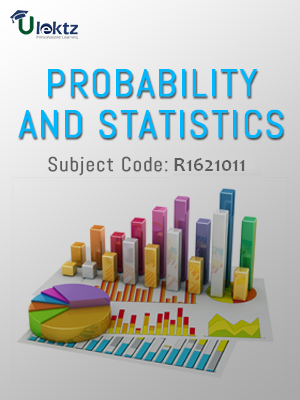uLektz apps

•My WalletMy Order
•My Profile
•My Connections
•My Books
•My Videos
•My Tests
•My Calender
•My Messages
•My Shopping Cart
•My Orders
•Account Settings
•Help

uLektz apps

# Book Details# Probability and Statistics

Course Code:R1621011

Author:uLektz

University:

Regulation:2016

Categories:Civil

Format :ePUB3 (DRM Protected)

Type :eBook

FREE

Description :Probability and Statistics of R1621011 covers the latest syllabus prescribed by JNTU Kakinada for regulation 2016. Author: uLektz, Published by uLektz Learning Solutions Private Limited.

Note : No printed book. Only ebook. Access eBook using uLektz apps for Android, iOS and Windows Desktop PC.

##### Topics
###### UNIT I DISCRETE RANDOM VARIABLES AND DISTRIBUTIONS

1.1 Introduction - Random variables - Discrete random variable - Distribution function - Expectation

1.2 Moment generating functions-Moments and properties

1.3 Discrete distributions: Binomial, Poisson and geometric distributions and their fitting to data

###### UNIT II CONTINUOUS RANDOM VARIABLE AND DISTRIBUTIONS

2.1 Introduction-Continuous random variable-Distribution function-Expectation

2.2 Moment generating function-Moments and properties-Continuous distribution: Uniform, exponential and normal distribution, normal approximation to binomial distribution -Weibull, gamma distribution

###### UNIT III SAMPLING THEORY

3.1 Introduction - Population and samples

3.2 Sampling distribution of mean (σknown)

3.3 Central limit theorem-t-distribution- Sampling distribution of means ( σ unknown)- Sampling distribution of variances -χ2 and F-distributions- Point estimation- Maximum error of estimate - Interval estimation

###### UNIT IV TESTS OF HYPOTHESIS

4.1 Introduction - Hypothesis - Null and alternative hypothesis - Type I and Type II errors - Level of significance

4.2 One tail and two-tail tests - Tests concerning one mean and proportion

4.3 Two means - Proportions and their differences - ANOVA for one-way and two-way classified data

###### UNIT V CURVE FITTING AND CORRELATION

5.1 Introduction - Fitting a straight line

5.2 Second degree curve - exponential curve - power curve by method of least squares - Goodness of fit

5.3 Correlation and regression - Properties

###### UNIT VI STATISTICAL QUALITY CONTROL METHODS

6.1 Introduction - Methods for preparing control charts

6.2 Problems using x-bar, p, R charts and attribute charts

### Related Books